Test: Gaseous State - 1

# Test: Gaseous State - 1

Test Description

## 20 Questions MCQ Test Physical Chemistry | Test: Gaseous State - 1

Test: Gaseous State - 1 for Chemistry 2023 is part of Physical Chemistry preparation. The Test: Gaseous State - 1 questions and answers have been prepared according to the Chemistry exam syllabus.The Test: Gaseous State - 1 MCQs are made for Chemistry 2023 Exam. Find important definitions, questions, notes, meanings, examples, exercises, MCQs and online tests for Test: Gaseous State - 1 below.
Solutions of Test: Gaseous State - 1 questions in English are available as part of our Physical Chemistry for Chemistry & Test: Gaseous State - 1 solutions in Hindi for Physical Chemistry course. Download more important topics, notes, lectures and mock test series for Chemistry Exam by signing up for free. Attempt Test: Gaseous State - 1 | 20 questions in 40 minutes | Mock test for Chemistry preparation | Free important questions MCQ to study Physical Chemistry for Chemistry Exam | Download free PDF with solutions
 1 Crore+ students have signed up on EduRev. Have you?
Test: Gaseous State - 1 - Question 1

### The ratio of root mean square velocity to average velocity of a gas molecule at a particular temperature is:

Detailed Solution for Test: Gaseous State - 1 - Question 1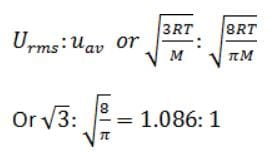Test: Gaseous State - 1 - Question 2

### The temperature at which a real gas obeys the ideal gas laws over a wide range of pressure is:

Detailed Solution for Test: Gaseous State - 1 - Question 2
• The temperature at which the real or non-ideal gas behaves as an ideal gas over a wide range of pressure is known as Boyle temperature.
• Boyle temperature (TB) is related to the Vander Waal’s constant a, b as given below. At this temperature, the attractive and repulsive forces acting on the gas particles arrive at a balance for a real gas.
• TB = a/Rb
Test: Gaseous State - 1 - Question 3

### Equal weights of methane and oxygen and mixed in an empty container at 25°C. The fraction of the total pressure exerted by oxygen is:

Detailed Solution for Test: Gaseous State - 1 - Question 3

Equal masses of methane and oxygen are mixed in an empty container at 25oC. The fraction of the total pressure exerted by oxygen is 1/3.

• Let 32 g of oxygen are mixed with 32 g of methane.
• The molar masses of methane and oxygen are 16 g/mol and 32 g/mole respectively.
• The number of moles of methane = 32g/16g ​=2 moles.
• The number of moles of oxygen = 32g/32g​ =1 mole.
• Total number of moles =2+1=3.

• The mole fraction of oxygen  =1/1+2​= 1/3

• The mole fraction is proportional to the fraction of the total pressure exerted.

• Hence, the fraction of the total pressure exerted by oxygen is 1/3

Test: Gaseous State - 1 - Question 4

Helium atom is two times heavier than a hydrogen molecule. At 298 K, the average kinetic energy of a helium atom is:

Detailed Solution for Test: Gaseous State - 1 - Question 4

Kinetic energy of gas molecule depends on temperature and does not depend upon the nature of gas.

K.E =(3/2)kT.

Thus hydrogen and helium have same K.E at same temperature

Test: Gaseous State - 1 - Question 5

No cooling occurs, when an ideal gas undergoes unrestrained expansion, because the molecules:

Detailed Solution for Test: Gaseous State - 1 - Question 5

Ideal gas has no attractive force between the particles

Test: Gaseous State - 1 - Question 6

A liquid is in equilibrium with its vapour at it’s boiling point. On the average, the molecules in the two phases have equal:

Detailed Solution for Test: Gaseous State - 1 - Question 6

When a liquid is in equilibrium with its vapout at its boiling point, the molecules in the two phases have equal kinetic energy.

At equilibrium, the rate of molecules going from the liquid to the gaseous phase equal to the rate of molecules going from the gaseous phase to the liquid surface and hence they have equal kinetic energy.

Test: Gaseous State - 1 - Question 7

Rate of diffusion of a gas is:

Detailed Solution for Test: Gaseous State - 1 - Question 7

Graham's law states that the rate of diffusion or of effusion of a gas is inversely proportional to the square root of its molecular weight.

Rate of Diffusion is proportional to 1/ √M

Test: Gaseous State - 1 - Question 8

The average velocity of an ideal gas molecule  at 27°C is 0.3 m/s. The average velocity at 927°C will be

Detailed Solution for Test: Gaseous State - 1 - Question 8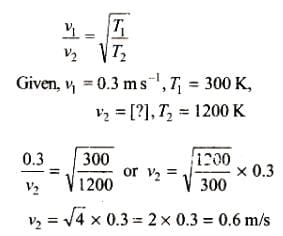Test: Gaseous State - 1 - Question 9

In van der Waals’ equation of state for a non-ideal gas, the term that accounts for intermolecular forces is:

Detailed Solution for Test: Gaseous State - 1 - Question 9

In van der Waals’ equation of state:
(p + a/V2) (V - b) = RT (for 1 mole)

The first factor (p + a/V2) correct for intermolecular force while the second term (V - b) correct for molecular volume.

Test: Gaseous State - 1 - Question 10

A bottle of dry ammonia and a bottle of dry hydrogen chloride connected through a long tube are opened simultaneously at both ends the white ammonium chloride ring first formed will be:

Detailed Solution for Test: Gaseous State - 1 - Question 10
• According to Graham's law of effusion:
Rate of effusion = √(1/Molar mass)
i.e. Gas of higher molar mass will effuse slowly, therefore, move a shorter distance.
• NH3 molar mass is 17 and HCl molar mass is 36.5. So, HCl effuses slowly and the formation of NH4Cl white rings are formed near the HCl
Test: Gaseous State - 1 - Question 11

The value of van der Waals’ constant ‘a’ for the gases O2, N2, NH3 and CH4 are 1.360, 1.390, 4.170 and 2.253 L2 atm mol–2 respectively. The gas which can most easily be liquefied is:

Detailed Solution for Test: Gaseous State - 1 - Question 11
• The ease of liquification of a gas depends on their intermolecular force of attraction which in turn is measured in terms of van der Waals’ constant ‘a’.
• Hence, the higher the value of ‘a’, the greater the intermolecular force of attraction, the easier the liquification.
• In the present case, NH3 has the highest ‘a’, can most easily be liquefied.
Test: Gaseous State - 1 - Question 12

The density of neon will be highest at:

Detailed Solution for Test: Gaseous State - 1 - Question 12
• According to the ideal gas equation:
P = dRT/M i.e. d = PM/RT
• As per the above equation at high pressure and low-temperature, density is maximum.
Test: Gaseous State - 1 - Question 13

The rate of diffusion of methane at a given temperature is twice that of a gas X. The molecular weight of X is:

Detailed Solution for Test: Gaseous State - 1 - Question 13

The rate of diffusion of methane at a given temperature is twice that of gas X i.e. rCH4​ ​= 2rX​
∵ The rate of diffusion is inversely proportional to the square root of its molar mass.
Therefore,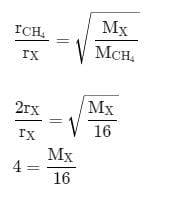Thus, molecular mass of gas X is M​= 64.0  g/mol

Test: Gaseous State - 1 - Question 14

According to kinetics theory of gases, for a diatomic molecule:

Detailed Solution for Test: Gaseous State - 1 - Question 14

As we know: KE = (3/2) ​RT
KE ∝ T
i.e. mean transitional kinetic energy of the molecules is proportional to the absolute temperature.

Test: Gaseous State - 1 - Question 15

At constant volume, for a fixed number of moles of a gas the pressure of the gas increases with rise of temperature due to:

Test: Gaseous State - 1 - Question 16

Equal weights of ethane and hydrogen are mixed in an empty container at 25°C. The fraction of the total pressure exerted by hydrogen is:

Detailed Solution for Test: Gaseous State - 1 - Question 16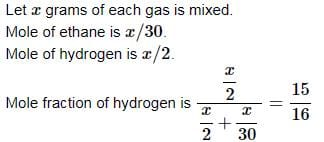Partial pressure of hydrogen/total pressure = mole fraction of hydrogen =15:16

Test: Gaseous State - 1 - Question 17

The ratio between the root mean square speed of H2 at 50 K and that of O2 at 800 K is:

Detailed Solution for Test: Gaseous State - 1 - Question 17

The rms velocity is directly proportional to the square root of temperature and inversely proportional to the square root of molecular weight.

The ratio between the root mean square velocity of H2​ at 50K and that of O2​ at 800K is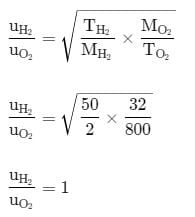Test: Gaseous State - 1 - Question 18

The compressibility factor for an ideal gas is:

Detailed Solution for Test: Gaseous State - 1 - Question 18

The deviation of ideal behaviour is introduced by compressibility factor Z.
i.e. Z = PV/nRT
for ideal gas PV = nRT, therefore, Z is 1.

Test: Gaseous State - 1 - Question 19

The critical temperature of water is higher than that of O2 because the H2O molecule has:

Test: Gaseous State - 1 - Question 20

According to Graham’s law, at a given temperature the ratio of the rates of diffusion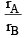of gases A and B is given by (where, p and M are pressure and molecular weights of gases A and B respectively)

Detailed Solution for Test: Gaseous State - 1 - Question 20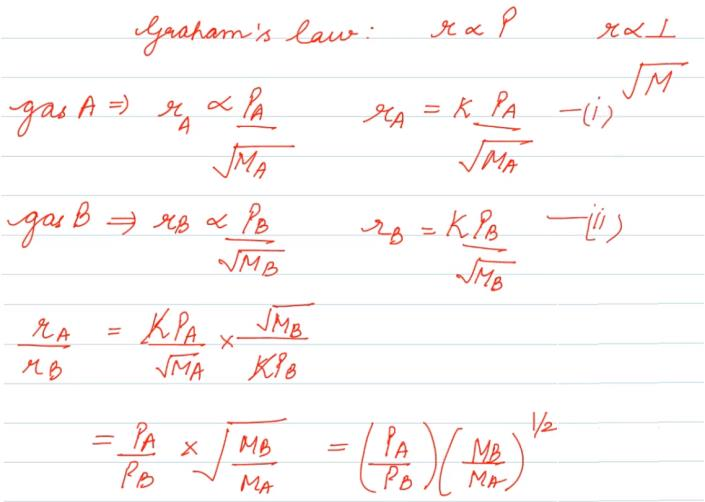Hence C is correct.

## Physical Chemistry

85 videos|131 docs|67 tests
 Use Code STAYHOME200 and get INR 200 additional OFF Use Coupon Code
Information about Test: Gaseous State - 1 Page
In this test you can find the Exam questions for Test: Gaseous State - 1 solved & explained in the simplest way possible. Besides giving Questions and answers for Test: Gaseous State - 1, EduRev gives you an ample number of Online tests for practice

## Physical Chemistry

85 videos|131 docs|67 tests